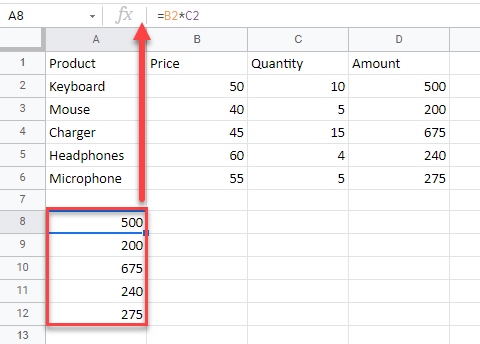# How to Copy and Paste Exact Formula in Excel & Google Sheets

This tutorial demonstrates how to copy and paste exact formulas in Excel and Google Sheets.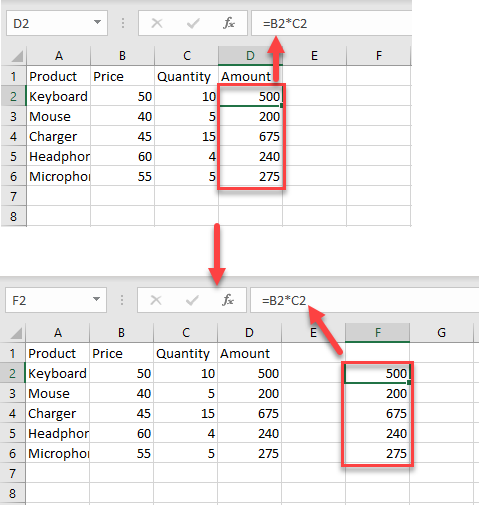## Copy and Paste Exact Formula – Find & Replace Feature

In the example below, you have an amount in Column D that is the product of values in Columns B and C. Now, say you want to copy the range (D2:D6) to another location, keeping the formulas, and without changing the cell references.

1. In cell D2, enter the formula:
``=B2*C2``

and drag it to the end of the range (D6).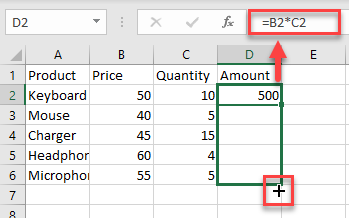1. To copy and paste the exact formula without changing the cell references to another place in your sheet, you need to convert the formulas to text and then copy them. To do that, select the range with formulas you want to copy. Then, in the Ribbon, go to Home > Editing > Find & Select > Replace.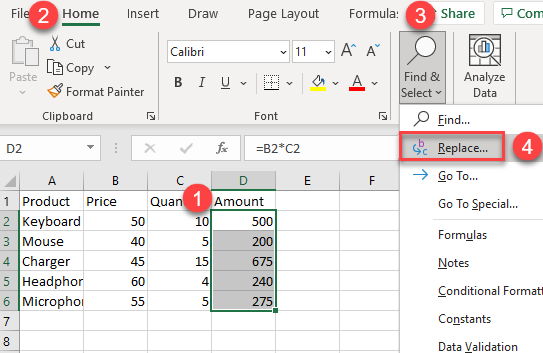1. The Find and Replace dialog box will appear. In the Replace with: box, type any symbol that you want to use instead of = (equal sign). Then click on the Replace All button.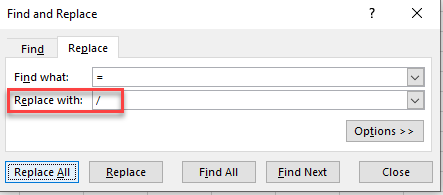1. As a result, all the equal signs in the range you selected are replaced with the chosen symbol. Since the content in each cell no longer starts with “=”, it will be in text form.
Now, select that range, right-click it, and from the drop-down menu, choose Copy (or use the CTRL + C shortcut).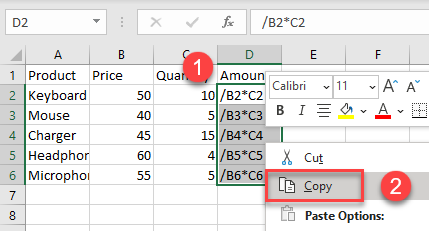1. Then, select the place where you want to paste the range, right-click it, and under Paste Options click on the Paste icon (or you could use the CTRL + V shortcut).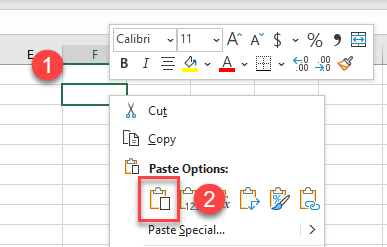1. This pastes the range in Column F.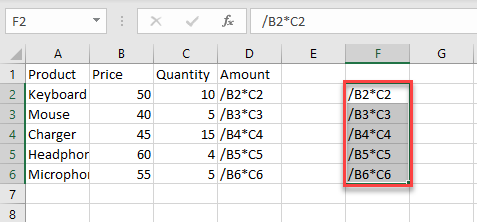1. To convert the copied and pasted ranges back to formulas, select both ranges and in the Ribbon, go to Home > Find & Replace > Replace.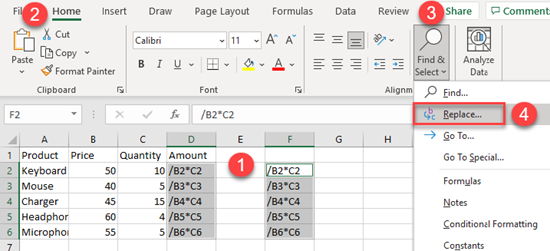1. In the Find and Replace dialog box, (1) under Find what: enter the symbol you chose in Step 3, and (2) under Replace with: enter = (an equal sign). Then, (3) click the Replace All button.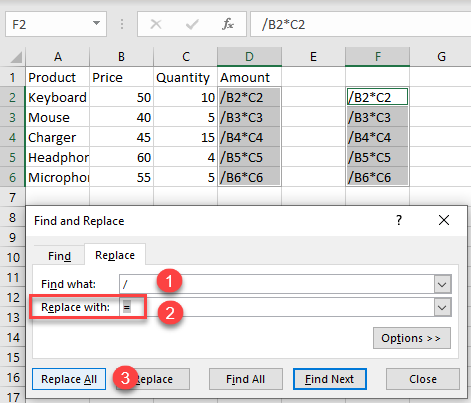1. After that, an information window will appear to inform you that all the replacements were made. To finish, just click OK.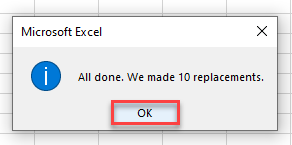As a result of Steps 1–9, you have the exact formula, copied from Column D and pasted to Column F, without changing cell references.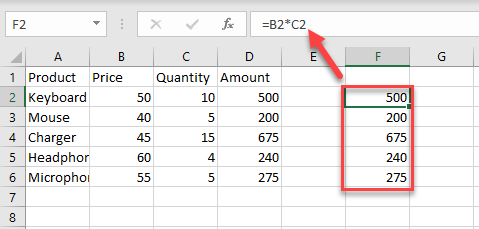You can also use a macro to copy and paste the exact formula in Excel.

## Copy and Paste Exact Formula – Absolute References

Another way to copy and paste a formula without changing references is to use absolute cell references. If you want a formula to consistently refer to a particular cell, regardless of where you copy or move that formula in the worksheet, you should use absolute cell references, which do not change when copied.

1. First, click on the cell where you want to enter a formula and type = (an equal sign) to begin. Then, select the cell you want to make an absolute reference and press F4:
``=\$E\$2*B2``

When done with the formula, press ENTER.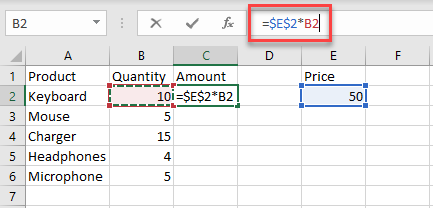1. Then, copy the formula by dragging it down the column.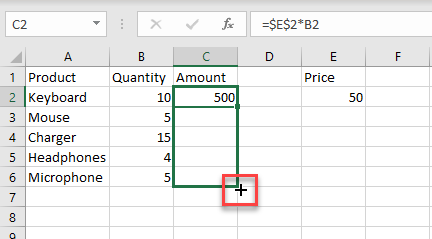As a result, the formula is copied down the column, and the reference to cell E2 does not change.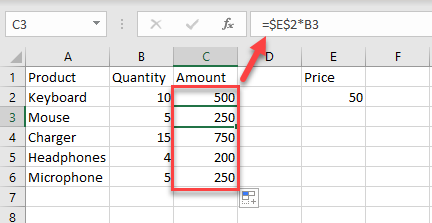## Copy and Paste Exact Formula in Google Sheets

In Google Sheets, you can copy and paste the exact formula using absolute references and dragging by performing the same steps shown above for Excel (or copy from Excel).

To copy the exact formula with the Find and Replace feature, the steps are a bit different.

1. In the cell D3, enter the formula:
``=B2*C2``

and drag the fill handle down the cells you want to fill with formulas (D2:D6).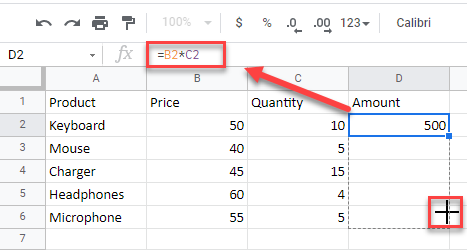1. After that, (1) select the range and in the Menu, (2) click on Edit. From the drop-down menu, (3) choose to Find and replace.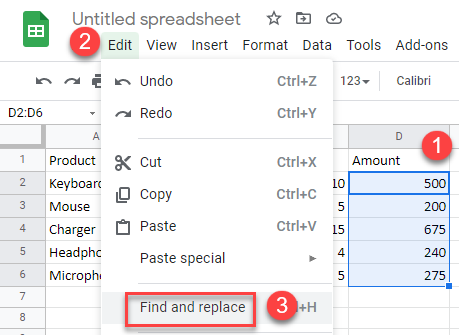1. The Find and Replace dialog box will appear. (1) In the Find box, type = (an equal sign); and (2) in the Replace with box, type the symbol you want to replace “=” with. (3) Then indicate the specific range where you want to do the replacement, and (4) check the box next to the Also search within formulas. Finally, (5) click the Replace all button and (6) click Done.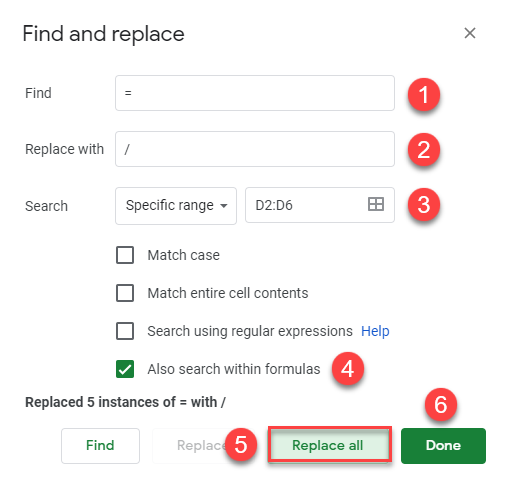1. As a result, all the = (equal signs) are replaced with the symbol you chose, and the range will no longer be read as formulas.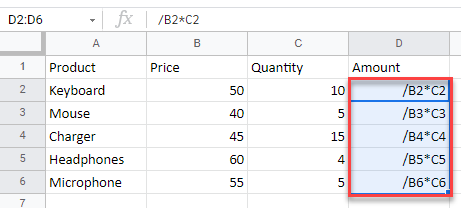1. Now, select that range (D2:D6), right-click it, and click on Copy (or use the CTRL + C shortcut).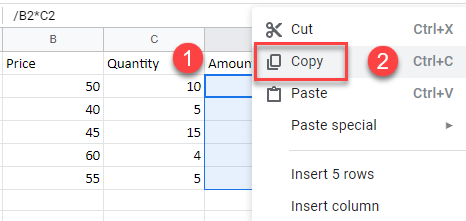1. Select the place where you want to paste the range, right-click it and click on Paste (or use the CTRL + V shortcut).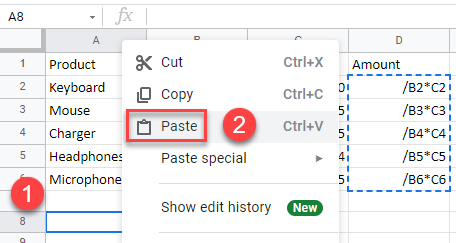1. This pastes the (text) range in A8:A12.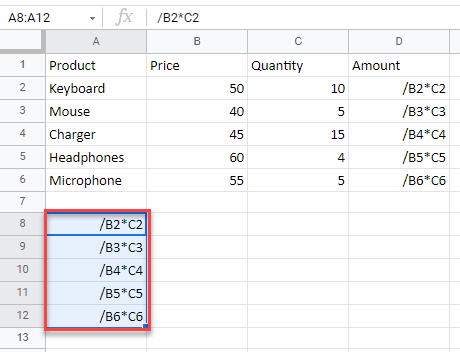1. To convert both the copied and pasted ranges back to formulas, , (1) select them and in the Menu, (2) click on Edit and (3) choose to Find and replace.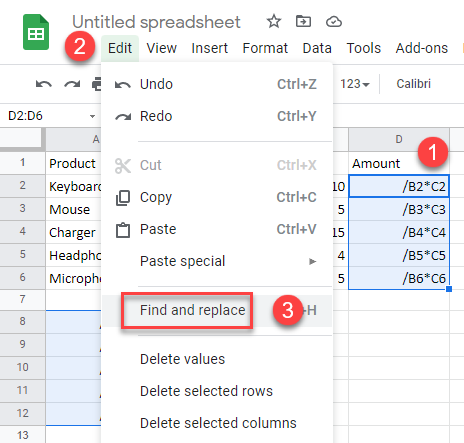1. The Find and replace window will appear. (1) In the Find box, type the symbol you used in Step 3 (in this example, slash). (2) In the Replace with box, type = (an equal sign). (3) Then, specify the scope for the replacement (in this example, “This sheet”), and (4) check the box next to Also search within formulas. Finally, (5) click the Replace all button and (6) click Done.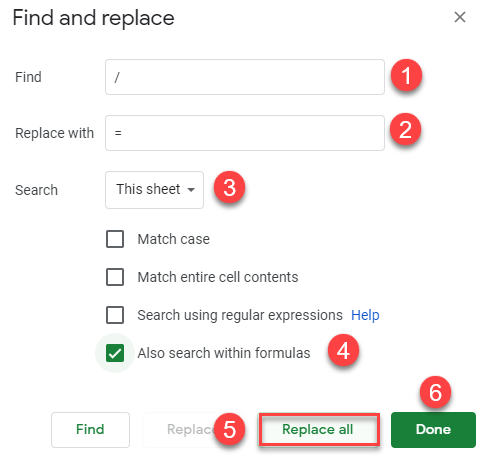As a result, you have the exact formula copied without changing cell references.